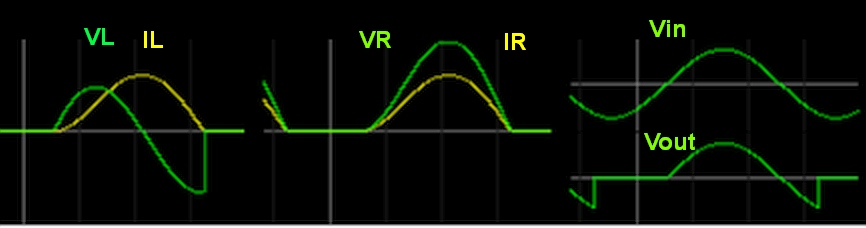# [SOLVED]Half wave rectifier with RL load

Status
Not open for further replies.

#### muheeb16

##### Member level 3why the current starts dropping at the circled point.Shouldn't it drop at 180 degree?

The applied voltage is well past its positive peak and is falling back towards zero.
The current starts to fall off too, but energy stored in the inductor delays the current peak, and the point where it finally droops.

It would drop at 180 degrees if there was no resistor, just a pure perfect inductor.

It would drop at 90 degrees (and follow the voltage) if there was just the resistor and no inductance.

With both, it drops somewhere between 90 and 180 degrees depending on how much R and how much L (in proportion).

•muheeb16

### muheeb16

Points: 2
but energy stored in the inductor delays the current peak, and the point where it finally droops.
That is what bugs me a lot.Can you elaborate it more?

The applied voltage is well past its positive peak and is falling back towards zero.
The current starts to fall off too, but energy stored in the inductor delays the current peak, and the point where it finally droops.

It would drop at 180 degrees if there was no resistor, just a pure perfect inductor.

It would drop at 90 degrees (and follow the voltage) if there was just the resistor and no inductance.

With both, it drops somewhere between 90 and 180 degrees depending on how much R and how much L (in proportion).

Actually the current would be in phase with only a resistive load (0 deg shift) and delayed 90 deg with only an inductor.

As the peak current is bit more than 45 deg phase delayed from voltage you can estimate the impedance of R is slightly less than L at this frequency.

180 deg phase shift max occurs with a minimum of a 2nd order effect or at least 2 reactive elements.
Here we only have a 1st order or one reactive element so the maximum phase shift can only be 90 deg.
The actual phase shift gives us the R/ X(f) vector ratios which = 1 at 45 deg. where X(f)=2pi*f*L

The waveforms demonstrate that the current also peaks when the voltage across R and L are equal.

That is what bugs me a lot.Can you elaborate it more?
When you apply a voltage across an inductor, the current ramps upward. The higher the voltage, the faster it ramps up.

So on the rising part of the sine wave the current also rises, and the rate of increase gets faster and faster as the voltage increases up to its peak.

Once the voltage peaks at 90 degrees, the current in the inductor will be ramping up at its fastest rate.

Even as the voltage starts to fall off, the current through the inductor continues to increase (because there is still a positive voltage across the inductor), but the rate of increase starts to slow down.

In fact the current will go on rising as long as there is ANY positive voltage across the inductor. The result is that peak inductor current will occur just as the voltage falls back to zero, right at the zero crossing point.

The maximum rate of current change will be right at the voltage peak, because the voltage across the inductor is highest.

Maximum peak inductor current occurs just as the voltage returns to zero.

The result is that the current through the inductor lags the applied sine wave voltage by ninety degrees.

•muheeb16

### muheeb16

Points: 2
1. The current is rectified by the diode.
2. Inductor voltage is always the derivative of current.
3. The fundamental sine wave voltage is zero at the peak of the current when the derivative=0. It is also 0V when the current =0 because the slope is 0.
4. No matter how complex the wave form, the fundamental voltage peak for an ideal inductor always leads the current peak by 90 degrees.Last edited by a moderator:

You said
"why the current starts dropping at the circled point.Shouldn't it drop at 180 degree?"
You are asking why isn't the slope VL at the input V zero crossing or Why isn't the "peak" shift" exactly 90 degrees between VS and VL on your top trace.

My simulation is the same as your example that shows that VL zero crossing is always at IL peak or in other words, the current peak lags 90deg from inductor voltage.

I showed both VL and Vout and Vin vs Vout across your R+L load.

Let's start as Vin is negative and the diode or CR (current rectifier) is reverse biased, Io and Vo=01. The Inductor integrates voltage and differentiates current
2. Vo rises with Vs at the same time due to the linear voltage rise on R (VR in sync with Vs and IR)
3. VL peaks when IL has the maximum +ve slope ( VL differentiates IL)
4. All the energy has been stored in L when IL reaches it's peak and then Vin goes negative as IL integrates back to zero with the stored energy.
5. But VL abruptly goes from negative to zero when the inductor runs out of stored energy as IL integrates back to zero.
6. If R was 0, then the VR=0 and the IL would be a complete sinewave shifted from 0V DC to some peak so IL would have been a full positive sinewave current shifted 90 from Vs. ( assuming Vs >> VD, diode drop voltage which tends to be <<1V)
7. Since power is lost across R, IL appears like a partly negative clipped sinewave shifted above the DC V=0 and the phase at which Vout returns from a negative sine to V=0 is an indication of the voltage drop and energy lost in R.

•muheeb16

Points: 2